Courses

# JEE(MAIN) Mathematics Mock Test - 3

## 30 Questions MCQ Test JEE Main Mock Test Series 2020 & Previous Year Papers | JEE(MAIN) Mathematics Mock Test - 3

Description
This mock test of JEE(MAIN) Mathematics Mock Test - 3 for JEE helps you for every JEE entrance exam. This contains 30 Multiple Choice Questions for JEE JEE(MAIN) Mathematics Mock Test - 3 (mcq) to study with solutions a complete question bank. The solved questions answers in this JEE(MAIN) Mathematics Mock Test - 3 quiz give you a good mix of easy questions and tough questions. JEE students definitely take this JEE(MAIN) Mathematics Mock Test - 3 exercise for a better result in the exam. You can find other JEE(MAIN) Mathematics Mock Test - 3 extra questions, long questions & short questions for JEE on EduRev as well by searching above.
QUESTION: 1

### if the pair of lines ax2 + 2hxy + by2 + 2gx + 2fy + c = 0

Solution: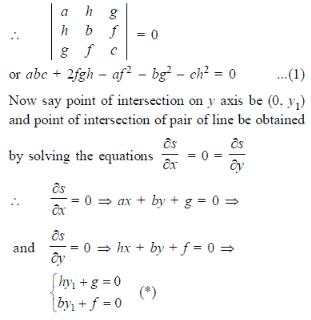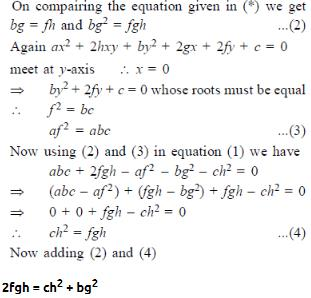QUESTION: 2

Solution:
QUESTION: 3

### The foci of the ellipse 25(x+1)2 + 9(y+2)2 = 225 are

Solution:QUESTION: 4

The product of all roots of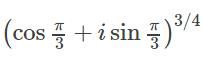is

Solution:
QUESTION: 5

In determinant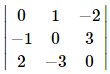radio of the cofactor of -3 and subdeterminant is

Solution:
QUESTION: 6

The differential equation of the family of lines passing through the origin is

Solution: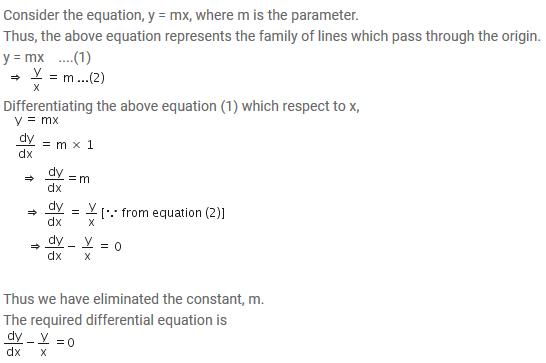QUESTION: 7
Log (x + y) - 2xy = 0, then y (0) =
Solution:
QUESTION: 8

If f: R → R and g : R → R defined by f(x) = 2x + 3 and g(x) = x2 + 7, then the value of x for which f(g(x)) = 25 are

Solution:
QUESTION: 9

The area bounded by the curve y = x2 - 4x, x-axis and line x = 2 is

Solution: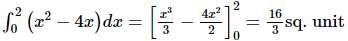QUESTION: 10

In the following question, a Statement of Assertion (A) is given followed by a corresponding Reason (R) just below it. Read the Statements carefully and mark the correct answer-
Assertion(A): f (x)   = log x3 and g (x)   = 3 log x are equal .
Reason(R) : Two functions f and g are said to be equal if their domains, ranges are equal and f (x)   = g (x) ∀ x in the domain .

Solution:
QUESTION: 11

Which of the following statements are true ?
(1) The amplitude of the product of complex numbers is equal to the product of their amplitudes.
(2) For any polynomial f(x) =0 with real co-efficients, imaginary roots occurs in conjugate paris.
(3) Order relation exists in complex numbers whereas it does not exist in real numbers.
(4) The value of ω used as a cube root of unity and as a fourth root of unity are different.

Solution:
QUESTION: 12

A tangent is drawn at the point (3√3 cos θ, sin θ) 0 < θ < (π/2) of an ellipse (x2/27) + (y2/1) = 1 the least value of the sum of the intercepts on the co-ordinate axes by this tangent is attained at θ =

Solution:
QUESTION: 13

In the following question, a Statement of Assertion (A) is given followed by a corresponding Reason (R) just below it. Read the Statements carefully and mark the correct answer-
Assertion (A):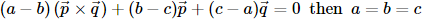Reason (R): The non zero vectors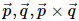are always linearly independent

Solution:
QUESTION: 14

How many numbers between 99 and 1000 can be formed from the digits 2,3,7,0,8,6 so that in each number each digit may occur once only?

Solution:
QUESTION: 15

If two dice are thrown, find the probability of getting an odd number of on one and multiple of 3 on the other is

Solution:
QUESTION: 16

The probabilities of solving a problem by three student A,B,C are 1/2, 1/3, 1/4 respectively. The probability that problem will be solved is

Solution:

We have, probability that A can solve the problem = P(A) = 1/2 ,
And in this way P(B) = 1/3 and P(C) = 1/4.
P(A cannot solve the problem) = 1 – P(A) = 1/2 ,
P(B cannot solve the problem) = 1 – P(B) = 1 – 1/3 = 2/3,
P(C cannot solve the problem) = 1 – P(C) = 1 – 1/4 = 3/4.
P(A, B, and C cannot solve the problem) = 1/2 x 2/3 x 3/4 = 1/4.
Therefore , P(Problem will be solve) = 1 – P(Problem is not solved by any of them)
= 1 – 1/4 = 3/4

QUESTION: 17

If the roots of ax2 + bx + c = 0 are α,β and roots of Ax2 + Bx + C = 0 are α + K, β + K, then B2 - 4AC/b2 - 4ac is equal to

Solution:
QUESTION: 18

Let f(x) be a polynominal function of second degree,If f(1) = f(-1) and a,b,c are in A.P., then f'(a),f'(b) and f'(c) are in

Solution:
QUESTION: 19

The total expenditure incurred by an industry under different heads is best presented as a

Solution:
QUESTION: 20
The distance of the point (2, 1, -1) from the plane x - 2y + 4z = 9 is
Solution:
QUESTION: 21
The orthocentre of a triangle whose vertices are [(2),((√3-1)/2)], ((1/2),-(1/2)) and (2,-(1/2)) is
Solution:
QUESTION: 22

If a line in the octant OXYZ and it makes equal angles with the axes, then

Solution:

In the octant OXYZ, all the three components are +ve...
now the line makes equal angles with all the axes,
therefore,
angle x = angle y= angle z
we know, cos²x + cos²y + cos²z = 1
thus, cos²x + cos²x + cos²x = 1
3cos²x = 1
cosx = 1/√3.... (since, cosx is +ve in first octant)
therefore..... l= m= n = cosx = cosy = cosz = 1/√3

QUESTION: 23

If sinα=-3/5, where π<α<(3π/2), then cos(α/2)=

Solution:
QUESTION: 24

The volume of a parallelopiped whose edges are -12i+αk, 3j-k and 2i+j-15k is 546, then the value of α is

Solution:
QUESTION: 25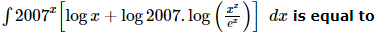Solution:
QUESTION: 26

An unknown polynomial yields a remainder of 2 upon division by x − 1, and a remainder of 1 upon division by x − 2. If this polynomial is divided by (x − 1)(x − 2), then the remainder is

Solution:
QUESTION: 27

If p , x1 , x2 , … xi … and q , y1 , y2 , … yi … are in A.P., with common difference a and b respectively, then the centre of mean position of the points Ai(xi, yi) where i = 1, 2, ..., n lies on the line

Solution:
QUESTION: 28

In the following question, a Statement of Assertion (A) is given followed by a corresponding Reason (R) just below it. Read the Statements carefully and mark the correct answer-
Assertion(A): f(x) = |x - 1| + |x - 2| + |x - 3|, where 2 < x < 3 is an identity function.
Reason(R): f : A → f(x) = x is identity function.

Solution:
QUESTION: 29

In the following question, a Statement of Assertion (A) is given followed by a corresponding Reason (R) just below it. Read the Statements carefully and mark the correct answer-
Assertion(A): A five digit number divisible by 3 is to be formed using the digits 0, 1, 2, 3, 4 and 5 with repetition. The total number formed are 216.
Reason(R) : If sum of any number is divisible by 3, then the number must be divisible by 3.

Solution:
QUESTION: 30

In the following question, a Statement of Assertion (A) is given followed by a corresponding Reason (R) just below it. Read the Statements carefully and mark the correct answer-
Assertion(A): The number of non-negative integral solutions of x1 + x2 + x3 + x4 ≤ 4 (where n is a +ve integer) is n+4C4.
Reason(R): The number of non-negative integral solutions of x1 + x2 + .... + xr = n is equal to n+r-1Cr.

Solution: